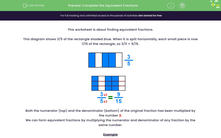# Complete the Equivalent Fractions

In this worksheet, students will fill in the gaps to create equivalent fractions.Key stage:  KS 2

Curriculum topic:   Number: Fractions, Decimals and Percentages

Curriculum subtopic:   Identify Equivalent Fractions

Popular topics:   Fractions worksheets, Equivalent Fractions worksheets

Difficulty level:#### Worksheet Overview

This activity is about finding equivalent fractions.

This diagram shows 3/5 of the rectangle shaded blue. When it is split horizontally, each small piece is now 1/15 of the rectangle, so 3/5 = 9/15.Both the numerator (top) and the denominator (bottom) of the original fraction have been multiplied by the number 3.

We can form equivalent fractions by multiplying the numerator and denominator of any fraction by the same number.

Example

Find the missing equivalent fractions.

 35
=
 610
=
9A
=
 1220

 35
=
 610
=
915
=
 1220

Are you ready to have a go at finding equivalent fractions?### What is EdPlace?

We're your National Curriculum aligned online education content provider helping each child succeed in English, maths and science from year 1 to GCSE. With an EdPlace account you’ll be able to track and measure progress, helping each child achieve their best. We build confidence and attainment by personalising each child’s learning at a level that suits them.

Get started